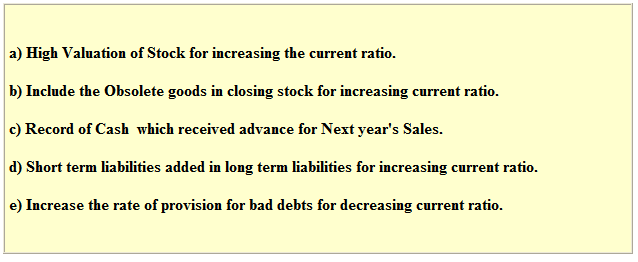222

## Balance Sheet \$type=three\$count=6\$author=hide\$comment=hide\$label=hide\$date=hide\$show=home\$s=0

In current ratio analysis, we will explain, how will current ratio affect to our business decisions. But before this, we will explain the simple meaning of current ratio.

Current ratio is a relationship between current assets and current liabilities. Just dividing current assets with current liabilities, we can calculate current ratio. Suppose, current assets are Rs. 250000 and current liabilities are Rs. 10000. Result will be 2.: 1. It means we have 2.5 times more current assets than current liabilities. In current assets, we can include cash, bank, prepaid expenses, marketable investment, sundry debtors, bill receivables and inventories.

In current liabilities, we can include outstanding expenses, sundry creditors, bank overdraft, short term taken loans and advances. Now, we analyze current ratio.

Main Point of Analysis

1. Rule of Thumb of Current Ratio 2:1

As a rule of thumb, current ratio should be 2:1. Proportion of current assets in current ratio should not be very high or very low than what has been given in the rule of thumb.

2.  If current ratio is less than 2:1

If current ratio is less than 2:1, then it means we have no sufficient current assets to pay our current liabilities. This will not show a good short term position. So, our current ratio must be 2:1.

3.  If current ratio is more  than 2:1

If our current ratio is more than 2:1, it means, we are misusing our current assets. Suppose, our current ratio is 5:1. This is because of more Rs. 100000 cash in hand. But there is no investment use of this cash. Suppose, we have more 400000 what is necessary for minimum reserve but this bank balance will giving just saving interest earning if we have saving account in bank. But, if we invest this money in business, this money can give us high return. It may be 20% or more. Like this, if we have very high level of debtors. More debtors means more risk of bad debt. If we have high level of inventory, it will increase our cost of storing that more inventory. More inventory in our stock will also increase the risk of obsolescence loss.

4. Analysis of Exceptions of Rule of Thumb

Sometime, we may keep our current ratio more or less than rule of thumb that is 2:1. But before taking this decision, we have to analysis important factors:

a) Good Reputation

If company's reputation in market is very good. At that time, company can operate with lower current ratio because company can sell his product on credit at high level and creditor may give loan for long period.

b) Seasonal Influence

Some current assets may be seasonal. At that time, current ratio may be high at that season. For example, at Diwali, sweet shops buy high quantity of raw material because at that time, it is expected that sale will be very high.

5. Analysis of Limitation of Current Ratio

If you are creditor or any other interested party, you should not only analyze above points of current ratio, but you should also analyze the limitation of current ratio. One of limitation of window dressing. For changing the current ratio, company may change the value of current assets or current liabilities.

Following things may happen.

Related : Introduction of Ratio AnalysisName

ltr
item
Accounting Education: Current Ratio Analysis
Current Ratio Analysis
http://3.bp.blogspot.com/-sKrON6STxfs/TpvCLd4wBAI/AAAAAAAAG6M/6_5kP1Nj82w/s640/limitation+of+ratio.PNG
http://3.bp.blogspot.com/-sKrON6STxfs/TpvCLd4wBAI/AAAAAAAAG6M/6_5kP1Nj82w/s72-c/limitation+of+ratio.PNG
Accounting Education
https://www.svtuition.org/2011/10/current-ratio-analysis.html
https://www.svtuition.org/
https://www.svtuition.org/
https://www.svtuition.org/2011/10/current-ratio-analysis.html
true
2410664366776677676
UTF-8